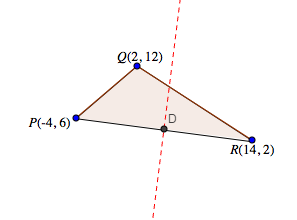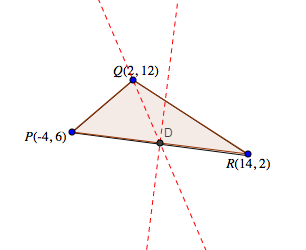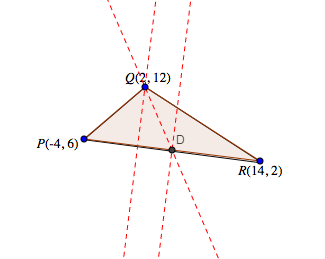### Home > PC > Chapter 6 > Lesson 6.1.4 > Problem6-63

6-63.
1. Using ΔPQR, find the equations for the following lines. Homework Help ✎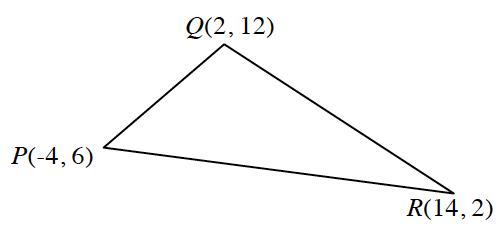1. The perpendicular bisector of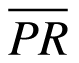.

2. The median line that goes from Q to the midpoint of.

3. The altitude of ΔPQR from Q to side.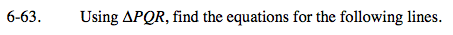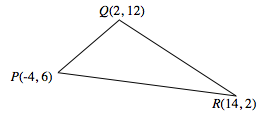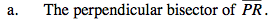1. Find the slope of the ⊥ line.
2. Find the midpoint (D)
3. Substitute into the point-slope form.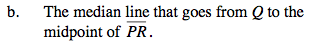1. Given the points D and Q, find the slope
2. Use the point-slope form of a line to find an equation.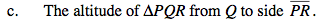1. Find the slope of the ⊥ line.
2. Then using the slope and pt. Q, use the form y = mx + b to find b.
3. Then substitute back m and b back into the form.

$y=\frac{9}{2}(x-2)+12$